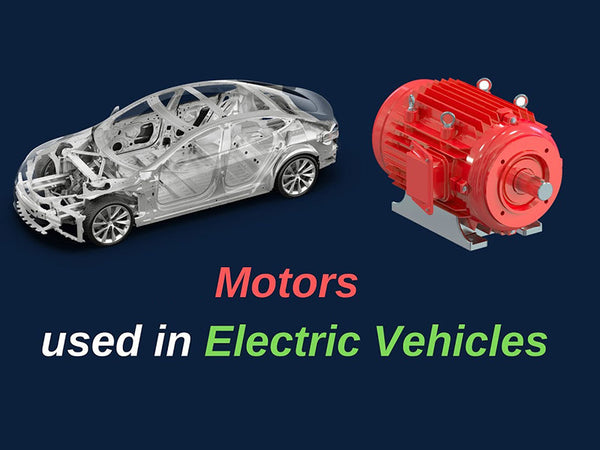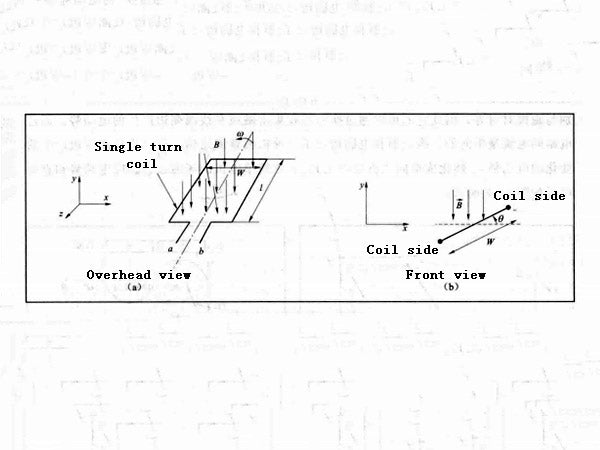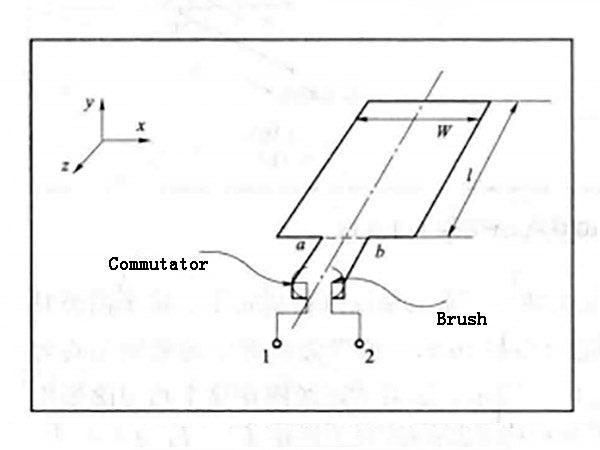An electric machine is an electromechanical device that can convert electrical energy into mechanical energy. When the vehicle is running, the motor can use the electrical energy provided by the energy source to transmit power and torque to the drive axle. When the vehicle is braking, the electric motor can also provide a reverse power flow process that converts the mechanical energy of the wheels into electrical energy. When a motor converts electrical energy into mechanical energy, it is called a motor; when a motor converts mechanical energy into electrical energy through a reverse power flow process, it is called a generator. The braking mode of the motor is called regenerative braking. There are electrical losses, mechanical losses and magnetic losses in the energy conversion process of the motor in both directions, which will affect the energy conversion efficiency of the motor. In any energy conversion process, some energy is lost. But compared to other types of energy conversion devices, motors have very high conversion efficiency.

In a pure electric vehicle, the electric motor is the only power unit; in a hybrid vehicle, the electric motor and the internal combustion engine are combined in series or parallel to power the vehicle. In pure electric and hybrid vehicles, the drive motor converts the electrical energy obtained from the energy storage device into mechanical energy to drive the wheels. Compared with the internal combustion engine, the advantage of the electric motor is that it can provide peak torque at low speed, and its instantaneous power rating can reach 2~3 times the rated power of the internal combustion engine. These features make motor-driven vehicles have more excellent acceleration performance.

Understand how voltage and torque are generated by studying a simple single-turn coil in a magnetic field. The magnetic field can be formed by a pair of magnetic poles, and the direction of the magnetic field is that the N pole points to the S pole, as shown in Figure 1(a). A single-turn coil is placed in the air gap between a pair of magnetic poles, allowing it to rotate freely around its axis of rotation under the action of a magnetic field. Pairs of poles and a single-turn coil form a simple motor that can be used to discuss the principles of electromechanical energy conversion. Another view of a single-turn coil under the action of a magnetic field is shown in Figure 1(b). A single-turn coil cuts magnetic field lines as it rotates about its axis of rotation.Figure 1 - Top view and front view of a single turn coil in a magnetic field

In the simple motor described above, the rotating single-turn coil is called the rotor, and the permanent magnets make up the stator. The magnetic flux in the stator pole pairs constitutes the magnetic field of the motor, also known as the field flux. The magnetic flux in the pole pair is assumed to be uniform, from the N pole to the S pole along the negative y-axis, as shown in Figure 1, if a single-turn coil rotates at a constant angular velocity ω in this uniform magnetic field, an induced voltage will be generated on both sides of the coil. The side of length l moving under the pole face is called the coil side. The side that connects the side of the coil at the bottom of the coil and has a length of W is called the end turn of the coil. The single-turn coil rotates at an angular velocity ω and forms an included angle θ with the direction of the magnetic field, as shown in Figure 1(b). The vector product (v×B) on the side of the coil is vBcosθ at any instant and is parallel to the side of the coil of length l. Therefore, the induced electromotive force on both sides of the coil is vBlcosθ. Since the vector product (v×B) on the base of the coil is always perpendicular to the base, the dot product with W is zero. No induced electromotive force is generated at the bottom of the coil, so the induced electromotive force generated by a single-turn coil is the sum of the induced electromotive force generated by the two sides

eab=2v·B·l·cosθ         (1-1)

Since the linear velocity can be represented by the angular velocity ω, that is, v=ω(W/2)=ωr, the induced electromotive force generated by the single-turn coil can be written as

eab=2B·l·r·ω·cosθ        (1-2)

The induced electromotive force can also be derived from a modified form of Faraday's law, the flux linkage of a single-turn coil is

Φ(θ)=-2B·l·r·sinθ         (1-3)

The maximum magnetic flux of the coil is

Φp=2B·l·r               (1-4)

The simple motor in Figure 1 produces a sinusoidal induced electromotive force when it rotates at a constant angular velocity in a uniform magnetic field. The changing electromotive force generated at the ab terminal of the simple motor of Figure 1 needs to be converted into a single-phase voltage by rectification to obtain a DC output. The output can be via commutators and brushes connected at the ends of the single-turn coil conductors (Figure 2). The commutator is connected to the end of the coil and rotates with the coil. The brushes are fixed and connected to external circuits. The positions of the two brushes are aligned with the pole pairs, so they can collect the electromotive force generated by the poles and the sides of the coil through the commutator. The current through the brushes is unidirectional. The commutator and brushes form a mechanical rectification structure, which can convert the changing electromotive force eab generated on the side of the coil into a unidirectional or DC voltage E12. The field flux, induced electromotive force and DC voltage of this simple motor are shown in Figure 3.Figure 2 - Commutator and brushes connected with wire diagramFigure 3 - Field flux, induced EMF and DC voltage for simple motor